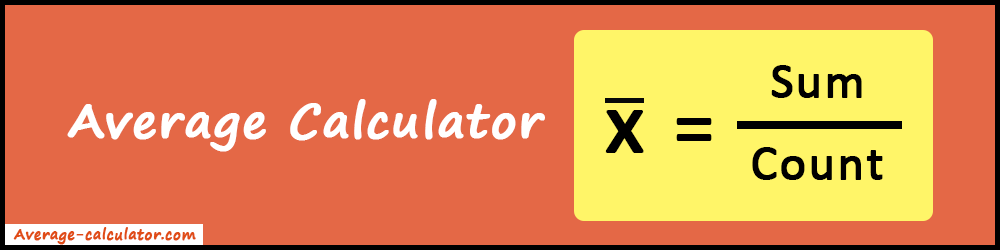# Average Calculator

Average Calculator allows you to find the mean or average of a set of numbers. It is also known as Mean Calculator.

Average calculation made easy and fast with our Average Calculator. It helps to calculate the mean of any set of data. Also, it's one of the best tools you can use for statistics calculation. In addition, it gives count, sum, geometric mean, root mean square, median, mode, range, and many more as an output.Get the assistance of the Mean Calculator whenever dealing with a large set of numbers. Hassle-free process and accurate results on time. Along with average calculation, it has many more features to show advanced values and calculation steps.

Do a thorough read of this article and know how to use the tool and how to find the average of a set of numbers using the average formula.

Firstly, let's understand what average is and how it is mathematically calculated. Whether you are a student, teacher, accountant, or anyone dealing with statistical work, this tool will be very useful for them.

### What is Average?

Average is a statistical concept. It technically means the arithmetic mean. The summation of all the numbers in a set divided by the total size of the set gives the average. An average number is a measure of 'central tendency'. However, there are many kinds of average numbers. For example, we have mean, median, mode and range. These are also used to determine central tendency.

#### Average Formula

Average =
 (Sum of all the numbers) (Count of all the numbers)

Note: Average and mean can be used interchangeably.

### How to Calculate the Average?

Let's take an example data set and try to find the average for it.

#### Example:

Suppose the data set is: 4, 7, 1, 6, 9, 11
Find the average of above set of numbers.

#### Solution:

Firstly, we will sum up all the data set values.
4 + 7 + 1 + 6 + 9 + 11 = 38

Total count = 6

So, according to the Average Formula,

Average =
 Sum Count
=
 38 6
=  6.333

Finally, the average is 6.333.

As you can see, the calculation is very simple. But it's simple for a small set of numbers. It becomes complicated when the data set is larger or the data set values are bigger. At that time, you can use our online average calculator to make this complex calculation easy, fast, and accurate. Also, it takes less than a second to give you the result. Isn't it awesome? So, keep our tool handy for any type of average or mean calculation.

#### Features of Average Calculator

• Very easy to use: Anyone can use the tool without any confusion. Because the user interface is made so simple. Also, we provide minimal and only necessary buttons to get the work done. All the things are clean and clear before you.
• Advanced values: Besides average calculation, you can also see some advanced values which might be helpful to you. Like sum, count, geometric mean, root mean square, median, mode, population standard deviation, sample standard deviation, smallest value, largest value, and range.
• Calculation steps: You can also see the calculation steps with the results. So, you can see and understand the mathematical calculation behind the process.
• Free tool: Our tool is free of cost. No hidden charges or subscription fees. It's completely free for anyone.
• Accurate results: Never expect an incorrect answer from our mean calculator. Pro-tip is that check your input data set before using the result.

#### Why use Mean Calculator?

The mean calculator is useful in many factors. For example, you can use it to calculate the average marks of your semester, the average price of the items, and the average rate of progressions. Use cases can be unlimited, but for all the needs our tool is ready to serve. Amazingly it's a reliable tool and simple to use. It gives the output in less than a second. Along with average, you get many more calculated advanced values. Undoubtedly, it stands as one of the best online tools for statistics. Even more, our calculator is fast, accurate, reliable, secure, and free to use. It also offers great cross-platform compatibility. You can use it anytime and anywhere. Instant average calculation at your fingertips.

### How to use the Average Calculator?

Before using the calculator, there are some pre-requisites you need to understand in order to use the online tool.

Input Format: Whatever be your data set, make sure that every number is separated by space or comma.

For Example: 4 6 9 1 12 5    OR    4, 6, 9, 1, 12, 5

This distinction is necessary in order to make the average calculator work. Whitespaces are ignored. So, no need to worry about them. You can keep it or remove it.

1. Firstly, enter the data set in proper format that is mentioned above.
2. After entering the input values just press the "Calculate" button.
3. As a result, you will get the average result with proper calculation steps.
4. In addition, our tool gives you some advanced values. Such as Median, Mode, Range, Geometric Mean, Root Mean Square, Count, Sum, Population Standard Deviation, Sample Standard Deviation, Smallest Value, and Largest Value.
5. If you wish to calculate the average for a different set, just use the "Reset" button to get a new start.